# RD Sharma Solutions Class 7 Chapter 15 Properties of Triangle

Read RD Sharma Solutions Class 7 Chapter 15 Properties of Triangle below, students should study RD Sharma class 7 Mathematics available on Studiestoday.com with solved questions and answers. These chapter wise answers for class 7 Mathematics have been prepared by teacher of Grade 7. These RD Sharma class 7 Solutions have been designed as per the latest NCERT syllabus for class 7 and if practiced thoroughly can help you to score good marks in standard 7 Mathematics class tests and examinations

Exercise 15.1

Question :1. Take three non-collinear points A. B and C on a page of your notebook. Join AB, BC and CA. What figure do you get? Name the triangle. Also, name

(i) The side opposite to ∠B

(ii) The angle opposite to side AB

(iii) The vertex opposite to side BC

(iv) The side opposite to vertex B.

Solution 1: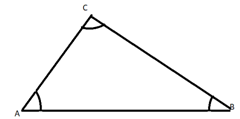(i) The side opposite to ∠B                          =  AC

(ii) The angle opposite to side AB              = ∠C

(iii) The vertex opposite to side BC           = A

(iv) The side opposite to vertex B             = AC

Question :2. Take three collinear points A, B and C on a page of your note book. Join AB. BC and CA. Is the figure a triangle? If not, why?

Solution  2: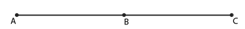The figure isn't a triangle at all. A triangle is a plane figure formed by three non-parallel line segments, according to description.

Question :3. Distinguish between a triangle and its triangular region.

Solution  3:

Triangle:

Three non- parallel line segments form a triangle, which is a plane figure.

Triangular region:

Its triangular area, on the other hand, includes the interior of the triangle as well as the triangle itself.

Question :4. D is a point on side BC of a △CAD is joined. Name all the triangles that you can observe in the figure. How many are they?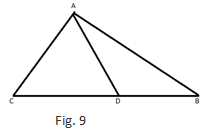Solution 4:

The following three triangles can be seen in the diagram.

△ ABC                  △ ACD                  △ ADB

Question :5. A, B, C and D are four points, and no three points are collinear. AC and BD intersect at O. There are eight triangles that you can observe. Name all the triangles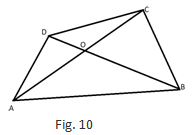Solution 5:

Given A, B, C and D are four points, and no three points are collinear

△ ABC                  △ ACD                  △ DBC

△ ABD                  △ AOB                  △ BOC

△ COD                  △ AOD

Question :6. What is the difference between a triangle and triangular region?

Solution  6:

Triangle:

Three non- parallel line segments form a triangle, which is a plane figure.

Triangular region:

Its triangular area, on the other hand, includes the interior of the triangle as well as the triangle itself.

Question :7. Explain the following terms:

(i) Triangle

(ii) Parts or elements of a triangle

(iii) Scalene triangle

(iv) Isosceles triangle

(v) Equilateral triangle

(vi) Acute triangle

(vii) Right triangle

(viii) Obtuse triangle

(ix) Interior of a triangle

(x) Exterior of a triangle

Solution 7:

(i) Triangle:

Three non- parallel line segments form a triangle, which is a plane figure.

(ii) Parts or elements of a triangle:

The three sides and the three angles of a triangle are together known as the parts or elements of that triangle.

(iii) Scalene triangle:

A scalene triangle is a triangle in which no two sides are equal.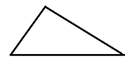(iv) Isosceles triangle:

An isosceles triangle is a triangle in which two sides are equal.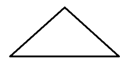(v) Equilateral triangle:

An equilateral triangle is a triangle in which all three sides are equal.(vi) Acute triangle:

An acute triangle is a triangle in which all the angles are less than 90o.(vii) Right triangle:

A right-angled triangle is a triangle in which one angle should be equal to 90o.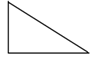(viii) Obtuse triangle:

An obtuse triangle is a triangle in which one angle is more than 90o.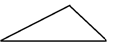(ix) Interior of a triangle:

Many of the points that are enclosed inside a triangle make up the triangle's interior.

(x) Exterior of a triangle:

All such points that are not enclosed within the triangle make up the triangle's exterior.

Question :8. In Fig. 11, the length (in cm) of each side has been indicated along the side. State for each triangle angle whether it is scalene, isosceles or equilateral:Solution 8:

(i)  It is a scalene triangle because no two sides are equal.

(ii)  It is an isosceles triangle because two of its sides, viz. PQ and PR, are equal.

(iii) It is an equilateral triangle because all its three sides are equal.

(iv)  It is a scalene triangle because no two sides are equal.

(v)  It is an isosceles triangle because two of its sides are equal.

Question :9. In Fig. 12, there are five triangles. The measures of some of their angles have been indicated. State for each triangle whether it is acute, right or obtuse.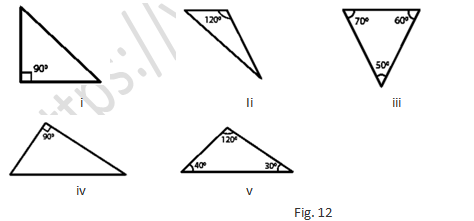Solution 9:

(i) It is a right triangle because one of its angles is 90o

(ii)  It is an obtuse triangle because one of its angles is 120o, which is greater than 90o

(iii)  It is an acute triangle because all its angles are less than 90o

(iv) It is a right triangle because one of its angle is 90o

(v) It is an obtuse triangle because one of its angle is 120o, which is greater than 90o.

Question :10. Fill in the blanks with the correct word/symbol to make it a true statement:

(i) A triangle has ……. sides.

(ii) A triangle has ……..vertices.

(iii) A triangle has ……..angles.

(iv) A triangle has ………parts.

(v) A triangle whose no two sides are equal is known as ………

(vi) A triangle whose two sides are equal is known as ……….

(vii) A triangle whose all the sides are equal is known as ………

(viii) A triangle whose one angle is a right angle is known as ……..

(ix) A triangle whose all the angles are of measure less than 90′ is known as ………

(x) A triangle whose one angle is more than 90′ is known as ……….

Solution 10:

(i) A triangle has three sides.

(ii) A triangle has three vertices.

(iii) A triangle has three angles.

(iv) A triangle has six parts.

(v) A triangle whose no two sides are equal is known as a scalene triangle.

(vi) A triangle whose two sides are equal is known as an isosceles triangle.

(vii) A triangle whose all the sides are equal is known as an equilateral triangle.

(viii) A triangle whose one angle is a right angle is known as a right triangle.

(ix) A triangle whose all the angles are of measure less than 90′ is known as an acute triangle.

(x) A triangle whose one angle is more than 90′ is known as an obtuse triangle.

Question :11. In each of the following, state if the statement is true (T) or false (F):

Solution   11:

(i) A triangle has three sides. True

(ii) A triangle may have four vertices.

Clarification:

Any three non-parallel line segments can make up a triangle. False

(iii) Any three line-segments make up a triangle.

Clarification:

Any three non-parallel line segments can make up a triangle. False

(iv) The interior of a triangle includes its vertices.

Clarification:

The interior of a triangle is the region enclosed by the triangle and the vertices are not enclosed by the triangle. False

(v) The triangular region includes the vertices of the corresponding triangle. True

(vi) The vertices of a triangle are three collinear points.

Clarification:

The vertices of a triangle are three non-collinear points. False

(vii) An equilateral triangle is isosceles also. True

(viii) Every right triangle is scalene.

Clarification:

A right triangle can also be an isosceles triangle. False

(ix) Each acute triangle is equilateral.

Clarification:

Each acute triangle is not an equilateral triangle, but each equilateral triangle is an acute triangle. False

(x) No isosceles triangle is obtuse.

Clarification:

An isosceles triangle can be an obtuse triangle, a right triangle or an acute triangle. False

Exercise 15.2

Question :1. Two angles of a triangle are of measures 150o and 30o. Find the measure of the third angle.

Solution   1:

Given: Two angles of a triangle 150o and 30o

Let x be the required third angle

As we know the total of all three angle of a triangle = 180o

So,150o + 30o + x = 180o

135o + x = 180o

x = 180o – 135o = 45o

Thus, the third angle is 45o

Question :2. One of the angles of a triangle is 130o, and the other two angles are equal. What is the measure of each of these equal angles?

Solution  2:

Given: in a triangle one of the angle is 130o, and the other two angles are equal.

So, let x be the second and third angle

As we know the total of all three angle of a triangle = 180o

130o + x + x = 180o

130o + 2x = 180o

2x = 180– 130o

2x = 50o

x = 50/2  = 25o

Thus, the two other angles are 25o each

Question :3. The three angles of a triangle are equal to one another. What is the measure of each of the angles?

Solution  3:

Given: all three angles are equal

So, let x be each angle

As we know the total of all three angle of a triangle = 180o

x + x + x = 180o

3x = 180o

x = 180/3 = 60o

Thus, angle is 60o each

Question :4. If the angles of a triangle are in the ratio 1: 2: 3, determine three angles.

Solution  4:

Given: The triangles’ angle are in the ratio of 1: 2: 3

So, we take angle first as x, angle second as 2x and angle third as 3x

As we know the total of all three angle of a triangle = 180o

x + 2x + 3x = 180o

6x = 180o

x = 180/6 = 30o

2x = 30o × 2 = 60o

3x = 30o × 3 = 90o

Thus, the angle first is 30o, angle second is 60o and angle third is 90o.

Question :5. The angles of a triangle are (x − 40)o, (x − 20)o and (1/2 − 10)o. Find the value of x.

Solution  5:

Given: Angles of a triangle are (x − 40)o, (x − 20)o and ( 1/2− 10)o.

As we know the total of all three angle of a triangle = 180o

(x − 40)o + (x − 20)o + ( 1/2 − 10)o = 180o

x + x + 1/2 – 40o – 20– 10o = 180o

x + x + 1/2 – 70o = 180o

5x/2 = 180+ 70o

5x/2 = 250o

x =  2/5 × 250o =  100o

So, the value of x is 100o

Question 6. The angles of a triangle are arranged in ascending order of magnitude. If the difference between two consecutive angles is 10o. Find the three angles.

Solution 6:

Let x be the angle first; angle second be x + 10o; angle third be x + 10o + 10o

As we know the total of all three angle of a triangle = 180o

x + x + 10o + x + 10o +10o = 180o

3x + 30 = 180

3x = 180 – 30

3x = 150

x = 150/3 = 50o

Angle first= 50o

Angle second = x + 10o = 50 + 10 = 60o

Angle third = x + 10o +10o = 50 + 10 + 10 = 70o

Question 7. Two angles of a triangle are equal and the third angle is greater than each of those angles by 30o. Determine all the angles of the triangle

Solution 7:

Let x be the first and second angle; according to the question third angle is greater than each of those angles by 30o

Thus, the third angle is greater than the first and second by 30o = x + 30o

The angles first and the second are equal

As we know the total of all three angle of a triangle = 180o

x + x + x + 30o = 180o

3x + 30 = 180

3x = 180 – 30

3x = 150

x =  150/3 = 50o

Angle third = x + 30o = 50o + 30o = 80o

The angle first and the second is 50o and the angle third is 80o.

Question 8. If one angle of a triangle is equal to the sum of the other two, show that the triangle is a right triangle.

Solution 8:

Let the measure of angles be m, n, o

Thus, can write above statement as m = n + o

m + n + o = 180o

Replacing the above value

m + m = 180o

2m = 180o

m = 180/2 = 90o

The given triangle is a right-angled triangle because one angle is 90o.

Question 9. If each angle of a triangle is less than the sum of the other two, show that the triangle is acute angled.

Solution  9:

Let the measure of angles be a, b and c

From the above statement we write as

a> b + c

b < a + c

c < a + b

Thus, triangle is an acute triangle

Question 10. In each of the following, the measures of three angles are given. State in which cases the angles can possibly be those of a triangle:

(i) 63o, 37o, 80o

(ii) 45o, 61o, 73o

(iii) 59o, 72o, 61o

(iv) 45o, 45o, 90o

(v) 30o, 20o, 125o

Solution 10:

(i) 63o + 37+ 80o = 180o

Angles form a triangle

(ii) 45o+ 61o+ 73o = 179o

179 ≠ 180o

Thus, not a triangle

(iii) 59o+ 72o+ 61o = 192o

192 ≠ 180o

Thus, not a triangle

(iv) 45o+ 45o+ 90o = 180o

Angles form a triangle

(v) 30o, 20o, 125o =175o

179 ≠ 180o

Thus, not a triangle

Question 11. The angles of a triangle are in the ratio 3: 4: 5. Find the smallest angle.

Solution 11:

Let 3x, 4x, 5x be the measure of the angles

As we know the total of all three angle of a triangle = 180o

3x + 4x + 5x = 180o

12x = 180o

x = 180/12 = 15o

Smallest angle = 3x

= 3 × 15o = 45o

Thus, smallest angle = 45o

Question 12. Two acute angles of a right triangle are equal. Find the two angles.

Solution 12:

A Right triangle: whose one of the angle is a right angle

Let x, x, 90o  be the measure of angle

x + x + 90o= 180o

2x = 90o

x = 90/2 = 45o

The measure of two angles are 45o and 45o

Question 13. One angle of a triangle is greater than the sum of the other two. What can you say about the measure of this angle? What type of a triangle is this?

Solution 13:

Let the measure of the angles be a, b, c

From the question we can write as

a > b + c;

b > a + c;

c > a + b

a or b or c > 90o which is obtuse

Thus, triangle is an obtuse angle

Question 14. In the six cornered figure, (fig. 20), AC, AD and AE are joined. Find ∠FAB + ∠ABC + ∠BCD + ∠CDE + ∠DEF + ∠EFA.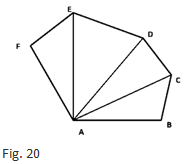Solution 14:

As we know the total of all three angle of a triangle = 180o

Thus, in ∆ABC,

∠CAB + ∠ABC + ∠BCA = 180- (i)

In△ ACD,

∠DAC + ∠ACD + ∠CDA = 180- (iii)

In △AEF,

∠FAE + ∠AEF + ∠EFA = 180o - (iv)

∠CAB + ∠ABC + ∠BCA + ∠DAC + ∠ACD + ∠CDA + ∠EAD + ∠ADE + ∠DEA + ∠FAE + ∠AEF +∠EFA = 720o

Thus, ∠FAB + ∠ABC + ∠BCD + ∠CDE + ∠DEF + ∠EFA = 720o

Question 15. Find x, y, z (whichever is required) from the figures (Fig. 21) given below:Solution 15:

∠ ADE = ∠ ABC [corresponding angles]

x = 40o

∠AED = ∠ACB                    [corresponding angles]

y = 30o

As we know the total of all three angle of a triangle = 180o

x + y + z = 180o (Angles of △ADE)

By putting the values

40o + 30o + z = 180o

z = 180o – 70o= 110o

Thus, we can find that the angles of the given triangle are x=40o, y=30o and z=110o

(ii)  As we can see that in △ADC, ∠ADC is equal to 90o.

As we know the total of all three angle of a triangle = 180o

45o + 90o + y = 180o (Sum of the angles of △ADC)

135o + y = 180o

y = 180o – 135o = 45o.

We can also say that in △ ABC, ∠ABC + ∠ACB + ∠BAC is equal to 180o.

(Sum of the angles of △ABC)

40o + y + (x + 45o) = 180o

40o + 45o + x + 45o = 180o (y = 45o)

x = 180o –130o

x = 50o

Thus, the required angles are 45o and 50o.

(iii) As we know the total of all three angle of a triangle = 180o

For △ABD:

∠ABD +∠ADB + ∠BAD = 180° (Sum of the angles of △ABD)

50o + x + 50o = 180o

100o + x = 180o

x = 180o – 100o = 80o

For △ ABC:

∠ABC + ∠ACB + ∠BAC = 180o (Sum of the angles of △ABC)

50o + z + (50o + 30o) = 180o

50o + z + 50o + 30o = 180o

z = 180o – 130o =50o

∠ADC + ∠ACD + ∠DAC = 180o (Sum of the angles of △ADC)

y + z + 30o = 180o

y + 50o + 30o = 180o (z = 50o)

y = 180o – 80o = 1000

Thus, the required angles are 80o, 50o and 100o.

y = 50o

, ∠AED = ∠ACB (Corresponding angles)

z = 40o

As we know the total of all three angle of a triangle = 180o

x + 50o + 40o = 180o (Angles of △ADE)

x = 180o – 90o = 90o

Thus, the required angles are 50o, 40o and 90o.

Question 16. If one angle of a triangle is 60o and the other two angles are in the ratio 1: 2, find the angles.

Solution 16:

Let x be the one of the other two angles,

Thus, the 2x will be the second one,

As we know the total of all three angle of a triangle = 180o

60o + x + 2x = 180o

3x = 180o – 60o

3x = 120o

x =  120/3 = 40o

2x = 2 × 40o

2x = 80o

Hence, The required angles are 40o and 80o.

Question 17. If one angle of a triangle is 100o and the other two angles are in the ratio 2: 3. Find the angles.

Solution 17:

Let 2x be one of the other two angle, the 3x will be second angle.

As we know the total of all three angle of a triangle = 180o

100o + 2x + 3x = 180o

5x = 180o – 100o

5x = 80o

x =  80/5 = 16

2x = 2 ×16

2x = 32o

3x = 3×16

3x = 48o

Thus, the required angles are 32o and 48o.

Question 18. In △ABC, if 3∠A = 4∠B = 6∠C, calculate the angles.

Solution 18:

According to the question the given triangle, 3∠A = 6∠C

∠A = 2∠C - (i)

According to the question the same triangle, 4∠B = 6∠C

∠B = (6/4)∠C- (ii)

As we know the total of all three angle of a triangle = 180o

Thus, ∠A + ∠B + ∠C = 180o (Angles of △ABC)- (iii)

By putting the values of ∠A and ∠B in equation (iii),

=2∠C +  (6/4)∠C + ∠C = 180o

=(18/4)∠C = 180o

∠C = 40o

From equation (i),

∠A = 2∠C = 2 × 40

∠A = 80o

From equation (ii),

∠B =  (6/4)∠C =  (6/4)× 40o

∠B = 60o

Therefore, the three angles of the given triangle are ∠A = 80o, ∠B = 60o, ∠C = 40o

Question 19. Is it possible to have a triangle, in which?

(i) Two of the angles are right?

(ii) Two of the angles are obtuse?

(iii) Two of the angles are acute?

(iv) Each angle is less than 60o?

(v) Each angle is greater than 60o?

(vi) Each angle is equal to 60o?

Solution 19:

(i) No, even if a triangle has two right angles, the triangle's third angle must be zero, which is not true.

(ii) No, since the number of a triangle's three angles is always 180o, as we know. If two obtuse angles are added together, their number exceeds 180o, which is impossible in a triangle.

(iii) Yes, two acute angles are possible in right triangles and acute triangles.

(iv) No, because if each angle is less than 60o, the number of all three angles is less than 180o, which is impossible in a triangle.

(v) No, because if each angle is less than 60o, the number of all three angles is less than 180o, which is impossible in a triangle.

(vi) Yes, if each triangle angle is 60 o, the total of all three angles would be 180 o, which is possible in the case of a triangle.

Question 20. In△ ABC, ∠A = 100o, AD bisects ∠A and AD ⊥ BC. Find ∠B

Solution 20:In the question given that in △ABC, ∠A = 100o

Consider △ABD

We know that the sum of all three angles of a triangle is 180o.

Thus,

∠ABD + ∠BAD + ∠ADB = 180o                     [Sum of angles of △ABD]

Or,

∠ABD + 50o + 90o = 180o

∠ABD =180o – 140o = 40o

Question 21. In △ABC, ∠A = 50o, ∠B = 70o and bisector of ∠C meets AB in D. Find the angles of the triangles ADC and BDC

Solution 21: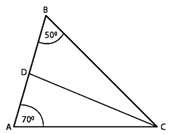As we know the total of all three angle of a triangle = 180o

For the given △ABC,

∠A + ∠B + ∠C = 180o       [Sum of angles of △ABC]

50o + 70o + ∠C = 180o

∠C= 180o –120o = 60o

∠ACD = ∠BCD =∠C2        [CD bisects ∠C and meets AB in D]

∠ACD = ∠BCD = 60/2 = 30o

According to the same way for the given △ACD,

∠DAC + ∠ACD + ∠ADC = 180o

50o + 30o + ∠ADC = 180o

∠ADC = 180o– 80o = 100o

According to the same way for the given △BCD,

∠DBC + ∠BCD + ∠BDC = 180o

70o + 30o + ∠BDC = 180o

∠BDC = 180o – 100o = 80o

Thus,

For △ADC= ∠A = 50o, ∠D = 100o ∠C = 30o

△BDC= ∠B = 70o, ∠D = 80o ∠C = 30o

Question 22: In ∆ABC, ∠A = 60o, ∠B = 80o, and the bisectors of ∠B and ∠C, meet at O. Find

(i) ∠C

(ii) ∠BOC

Solution 22:(i) As we know the total of all three angle of a triangle = 180o

For △ABC,

∠A + ∠B + ∠C = 180o                       [Sum of angles of ∆ABC]

60o + 80o + ∠C= 180o.

∠C = 180o – 140o = 40o.

(ii)For △OBC,

∠OBC = ∠B/2  = 80/2                               [OB bisects ∠B]

∠OBC = 40o

∠OCB = ∠C/2 = 40/2                    [OC bisects ∠C]

∠OCB = 20o

If we apply the above logic to this triangle,

∠OCB + ∠OBC + ∠BOC = 180o [Sum of angles of △OBC]

20o + 40o + ∠BOC = 180o

∠BOC = 180o – 60o = 120o

Question 23. The bisectors of the acute angles of a right triangle meet at O. Find the angle at O between the two bisectors.

Solution 23: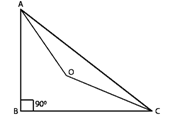As we know the total of all three angle of a triangle = 180o

For △ABC,

∠A + ∠B + ∠C = 180o

∠A + 90o + ∠C = 180o

∠A + ∠C = 180o – 90o

∠A + ∠C = 90o

For △OAC:

∠OAC =  ∠A/2                       [OA bisects ∠A]

∠OCA   = ∠C/2                          [OC bisects ∠C]

By applying same way to △OAC,

∠AOC + ∠OAC + ∠OCA = 180o                      [Sum of angles of △AOC]

∠AOC +  ∠A/2 +∠C/2    = 180o

∠AOC +  (∠A+∠C)/2 = 180o

∠AOC + 90/2 = 180o

∠AOC = 180o – 45o = 135o

Question 24.  In △ABC, ∠A = 50o and BC is produced to a point D. The bisectors of ∠ABC and ∠ACD meet at E. Find ∠E.

Solution 24: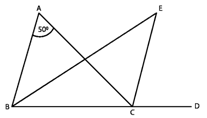∠ACD = ∠A + ∠B.               [Exterior angle is equal to the sum of two opposite interior angles]

As we know the total of all three angle of a triangle = 180o

∠ABC + ∠BCA + ∠CAB = 180o

∠A + ∠B + ∠BCA = 180o

∠BCA = 180o – (∠A + ∠B)

But EC bisects ∠ACD

Therefore ∠ECA = ∠ACD/2

∠ECA = (∠A + ∠B)/2 [∠ACD = (∠A + ∠B)]

But EB bisects ∠ABC

∠EBC =∠ABC/2 = ∠B/2

∠EBC = ∠ECA + ∠BCA

∠EBC =  (∠A + ∠B)/2 + 180o – (∠A + ∠B)

If we use same steps for △EBC,

∠B/2   + (∠A + ∠B)/2 + 180o – (∠A + ∠B) + ∠BEC = 180o

∠BEC =∠A + ∠B – (∠A + ∠B)/2 – ∠B/2

∠BEC = ∠A/2

∠BEC =  50/2 = 25o

Question 25. In ∆ABC, ∠B = 60°, ∠C = 40°, AL ⊥ BC and AD bisects ∠A such that L and D lie on side BC. Find ∠LAD

Solution 25:As we know the total of all three angle of a triangle = 180o

△ABC, we can write

∠A + ∠B + ∠C = 180o

∠A + 60o + 40o = 180o

∠A = 800

And  ∠DAC bisects ∠A

∠DAC = ∠A/2

∠DAC = 80o/2

Apply same steps for the △ADC,

As we know the total of all three angle of a triangle = 180o

∠ADC + ∠DCA + ∠DAC = 180o

∠ADC + 40o + 40o = 180o

∠ADC = 180– 80o = 1000

Exterior angle is equal to the sum of two interior opposite angles

But here AL perpendicular to BC

Question 26. Line segments AB and CD intersect at O such that AC ∥ DB. It ∠CAB = 35o and ∠CDB = 55o. Find ∠BOD.

Solution 26:AC parallel to BD and AB cuts AC and BD at A and B, respectively.

∠CAB = ∠DBA                     [Alternate interior angles]

∠DBA = 35o

As we know the total of all three angle of a triangle = 180o

For △OBD, we can say that:

∠DBO + ∠ODB + ∠BOD = 180°

35o + 55o + ∠BOD = 180o (∠DBO = ∠DBA and ∠ODB = ∠CDB)

∠BOD = 180o – 90o

∠BOD = 90o

Question 27. In Fig. 22, ∆ABC is right angled at A, Q and R are points on line BC and P is a point such that QP ∥ AC and RP ∥ AB. Find ∠PSolution 27:

AC parallel to QP and BR cuts AC and QP at C and Q, respectively.

∠QCA = ∠CQP                    [Alternate interior angles]

RP parallel to AB and BR cuts AB and RP at B and R, respectively,

∠ABC = ∠PRQ                     [Alternate interior angles]

As we know the total of all three angle of a triangle = 180o

For ∆ABC,

∠ABC + ∠ACB + ∠BAC = 180o

∠ABC + ∠ACB + 90o = 180o             [Right angled at A]

∠ABC + ∠ACB = 90o

Applying same way  for △PQR,

∠PQR + ∠PRQ + ∠QPR = 180o

∠ABC + ∠ACB + ∠QPR = 180o (∠ACB = ∠PQR and ∠ABC = ∠PRQ)

Or,

90o+ ∠QPR =180o (∠ABC+ ∠ACB = 90o

∠QPR = 90o

Exercise 15.3

Question 1. In Fig. 35, ∠CBX is an exterior angle of ∆ABC at B. Name

(ii) The interior opposite angles to exterior ∠CBX

Also, name the interior opposite angles to an exterior angle at A.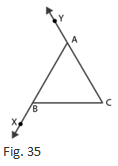Solution 1:

(i) The interior adjacent angle                                                    = ∠ABC

(ii) The interior opposite angles to exterior ∠CBX               = ∠BAC and ∠ACB

Also the interior angles opposite to exterior                        =∠ABC and ∠ACB

Question 2. In the fig. 36, two of the angles are indicated. What are the measures of ∠ACX and ∠ACB?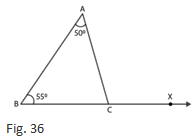Solution 2:

△ABC, ∠A = 50o and ∠B = 55o

As we know the total of all three angle of a triangle = 180o

∠A + ∠B + ∠C = 180o

50o+ 55o+ ∠C = 180o

∠C = 75o

∠ACB = 75o

∠ACX = 180o− ∠ACB = 180− 75o = 105o

Question 3. In a triangle, an exterior angle at a vertex is 95o and its one of the interior opposite angles is 55o. Find all the angles of the triangle.

Solution 3: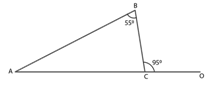The sum of interior opposite angles is equal to the exterior angle.

∠ABC+ ∠BAC = ∠BCO

55o + ∠BAC = 95o

∠BAC= 95o– 55o

∠BAC = 40o

As we know the total of all three angle of a triangle = 180o

For the given △ABC,

∠ABC + ∠BAC + ∠BCA = 180o

55o + 40o + ∠BCA = 180o

∠BCA = 180o –95o

∠BCA = 85o

Question 4. One of the exterior angles of a triangle is 80o, and the interior opposite angles are equal to each other. What is the measure of each of these two angles?

Solution 4:

Let us assume that P and Q are the two interior opposite angles.

We know that ∠P is equal to ∠Q

The sum of interior opposite angles is equal to the exterior angle.

Thus, from the figure,

∠P + ∠Q = 80o

∠P +∠P = 80o           [because ∠P = ∠Q]

2∠P = 80o

∠P =  80/2 =40o

∠P= ∠Q = 40o

Thus, each of the required angles is of 40o.

Question 5. The exterior angles, obtained on producing the base of a triangle both ways are 104o and 136o. Find all the angles of the triangle.

Solution 5:∠ABE and ∠ABC form a linear pair.

∠ABE + ∠ABC =180o

∠ABC = 180o– 136o

∠ABC = 44o

And, ∠ACD and ∠ACB form a linear pair.

∠ACD + ∠ACB = 180o

∠ACB = 180o– 104o

∠ACB = 76o

Sum of interior opposite angles is equal to the exterior angle.

Therefore, ∠BAC + ∠ABC = 104o

∠BAC = 104o – 44o = 60o

∠ACE = 76o and ∠BAC = 60o

Question 6. In Fig. 37, the sides BC, CA and BA of a △ABC have been produced to D, E and F respectively. If ∠ACD = 105o and ∠EAF = 45o; find all the angles of the △ABC.Solution 6:

△ABC, ∠BAC and ∠EAF    [vertically opposite angles]

Hence, ∠BAC = ∠EAF = 45o

Considering the exterior angle property,

∠BAC + ∠ABC = ∠ACD = 105o

By repositioning ∠ABC = 105o– 45o = 60o

As we know the total of all three angle of a triangle = 180o

∠ABC + ∠ACB +∠BAC = 180°

∠ACB = 75o

Thus, the angles are 45o, 60o and 75o.

Question 7. In Fig. 38, AC perpendicular to CE and C ∠A: ∠B: ∠C= 3: 2: 1. Find the value of ∠ECD.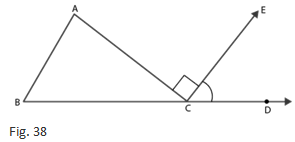Solution 7:

Let 3x, 2x and x be the angles of the triangle

As we know the total of all three angle of a triangle = 180o

3x + 2x + x = 180o

6x = 180o

x = 30o

Also, ∠ACB + ∠ACE + ∠ECD = 180o

x + 90o + ∠ECD = 180o (∠ACE = 90o)

According to question x = 30o

Thus, ∠ECD = 60o

Question 8. A student when asked to measure two exterior angles of △ABC observed that the exterior angles at A and B are of 103o and 74o respectively. Is this possible? Why or why not?

Solution 8:

As we know the total of all three angle of a triangle = 180o

Internal angle at A + External angle at A = 180o

Internal angle at A + 103o =180o

A = 77o

Internal angle at B + External angle at B = 180o

Internal angle at B + 74o = 180o

B = 106o

Sum of internal angles at A and B

= 77o + 106o = 183o

It means that the sum of internal angles at A and B is greater than 180o, which cannot be possible.

Question 9. In Fig.39, AD and CF are respectively perpendiculars to sides BC and AB of △ABC. If ∠FCD = 50o, find ∠BADSolution 9:

As we know the total of all three angle of a triangle = 180o

△FCB,

∠FCB + ∠CBF + ∠BFC = 180o

50o + ∠CBF + 90= 180o

∠CBF = 180o – 50o – 90o = 40o

Applying same way for △ABD,

∠ABD + ∠BDA + ∠BAD = 180o

∠BAD = 180o – 90o – 40o = 50o

Question 10. In Fig.40, measures of some angles are indicated. Find the value of x.Solution 10:

As we know the total of all three angle of a triangle = 180o

From the figure we have,

∠AED + 120o = 180o (Linear pair)

∠AED = 180o – 120o = 60o

As we know the total of all three angle of a triangle = 180o

∠ADE + ∠AED + ∠DAE = 180o

∠ADE = 180– 60– 30o = 90o

According to the given figure,

∠FDC + 90o = 180o                             [Linear pair]

∠FDC = 180– 90o = 90o

Applying same way for △CDF,

∠CDF + ∠DCF + ∠DFC = 180o

90o + ∠DCF + 60o = 180o

∠DCF = 180– 60– 90= 30o

∠DCF + x = 180o                                 [Linear pair]

30o + x = 180o

x = 180o – 30o = 150o

Question 11. In Fig. 41, ABC is a right triangle right angled at A. D lies on BA produced and DE perpendicular to BC intersecting AC at F. If ∠AFE = 130o, find

(i) ∠BDE

(ii) ∠BCA

(iii) ∠ABC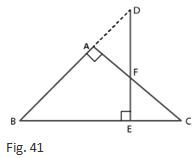Solution 11:

(i) ∠BAF + ∠FAD = 180o   [Linear pair]

∠FAD = 180– ∠BAF = 180– 90o = 90o

According to the figure,

∠ADF = 130o − 90o = 40o

∠BDE = 40o

(ii) As we know the total of all three angle of a triangle = 180o

For △BDE,

∠BDE + ∠BED + ∠DBE = 180o

∠DBE = 180– ∠BDE – ∠BED

∠DBE = 180– 40– 90= 50o- Equation (i)

According to the figure,

∠FAD = ∠ABC + ∠ACB                      [Exterior angle property]

90o = 50o + ∠ACB

∠ACB = 90– 50o = 40o

(iii) By the equation ∠ABC = ∠DBE = 50o

Question 12. ABC is a triangle in which ∠B = ∠C and ray AX bisects the exterior angle DAC. If ∠DAX = 70o. Find ∠ACB.

Solution 12: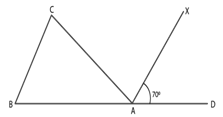In the question given information is that ABC is a triangle in which ∠B = ∠C

Also AX bisects the exterior angle DAC

∠CAX = ∠DAX                     [AX bisects ∠CAD]

∠CAX =70o                                         [given]

∠CAX +∠DAX + ∠CAB =180o

70o+ 70o + ∠CAB =180o

∠CAB =180o –140o =40o

∠ACB + ∠CBA + ∠CAB = 180o                        [Sum of the angles of ∆ABC]

∠ACB + ∠ACB+ 40o = 180o                              [∠C = ∠B]

2∠ACB = 180– 40o

∠ACB =  140/2 = 70o

Question 13. The side BC of △ABC is produced to a point D. The bisector of ∠A meets side BC in L. If ∠ABC= 30o and ∠ACD = 115o, find ∠ALCSolution 13:

In the question given information is that ∠ABC= 30o and ∠ACD = 115o

According to the figure,

∠ACD and ∠ACL                                 [linear pair]

∠ACD+ ∠ACB = 180o

115o + ∠ACB =180o

∠ACB = 180– 115o = 65o

As we know the total of all three angle of a triangle = 180o

Thus, for△ ABC,

∠ABC + ∠BAC + ∠ACB = 180o

30o + ∠BAC + 65o = 180o

∠BAC = 85o

∠LAC =  ∠BAC/2 = 85/2

Applying same way for △ALC,

∠ALC + ∠LAC + ∠ACL = 180o

∠ALC +  85/2 + 65o = 180o

∠ACL = ∠ACB

∠ALC = 180o – 85/2 – 65o

∠ALC = 72 ½o

Question 14. D is a point on the side BC of △ABC. A line PDQ through D, meets side AC in P and AB produced at Q. If ∠A = 80o, ∠ABC = 60o and ∠PDC = 15o, find

(i) ∠AQD

(ii) ∠APD

Solution 14:According to the figure

∠ABD and ∠QBD               [form a linear pair]

∠ABC + ∠QBC =180o

60o + ∠QBC = 180o

∠QBC = 120o

∠PDC = ∠BDQ                     [Vertically opposite angles]

∠BDQ = 15o

(i) △QBD:

∠QBD + ∠QDB + ∠BQD = 180o                      [Sum of angles of △QBD]

120o+ 15o + ∠BQD = 180o

∠BQD = 180– 135o

∠BQD = 45o

∠AQD = ∠BQD = 45o

(ii) △AQP:

∠QAP + ∠AQP + ∠APQ = 180o                      [Sum of angles of △AQP]

80o + 45o + ∠APQ = 180o

∠APQ = 55o

∠APD = ∠APQ = 55o

Question 15. Explain the concept of interior and exterior angles and in each of the figures given below. Find x and y (Fig. 42)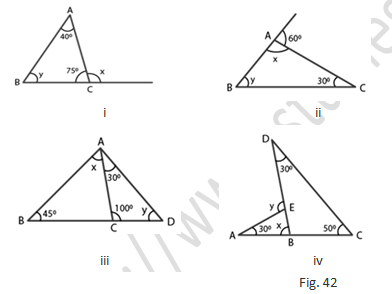Solution 15:

In a triangle if all the three angle elements inside the triangle then they are interior triangles.

The exterior angles are formed by extending the sides of a triangle, and if the side of a triangle is produced, the exterior angle so formed is equal to the sum of the two interior opposite angles.

(i) ∠ACB + x = 180o                           [Linear pair]

75o+ x = 180o

x = 105o

As we know the total of all three angle of a triangle = 180o

Thus, △ABC,

∠BAC+ ∠ABC +∠ACB = 180o

40o+ y +75o = 180o

y = 65o

(ii) x + 80= 180o                               [Linear pair]

x = 100o

In △ABC,

As we know the total of all three angle of a triangle = 180o

x + y + 30o = 180o

100o + 30o + y = 180o

y = 50o

(iii) As we know the total of all three angle of a triangle = 180o

Thus, for △ACD,

30o + 100o + y = 180o

y = 50o

Again, according to the figure

∠ACB + 100° = 180°

∠ACB = 80o

Applying same rule △ACB,

x + 45o + 80o = 180o

x = 55o

(iv) As we know the total of all three angle of a triangle = 180o

Thus, for △DBC,

30o + 50o + ∠DBC = 180o

∠DBC = 100o

According to the figure

x + ∠DBC = 180o                                 [Linear pair]

x = 80o

From the exterior angle property,

y = 30o + 80o = 110o

Question 16. Compute the value of x in each of the following figures:

Solution 16:(i) ∠ACD + ∠ACB = 180o                                  [linear pair]

By repositioning

∠ACB = 180– 112o = 68o

∠BAE + ∠BAC = 180o                                        [linear pair]

On rearranging we get,

∠BAC = 180o– 120o = 60o

As we know the total of all three angle of a triangle = 180o

Thus, for △ABC:

x + ∠BAC + ∠ACB = 180o

x = 180– 60– 68o = 52o

x = 52o

(ii) ∠ABC + 120o = 180o                    [linear pair]

∠ABC = 60o

Again, According to the figure we

∠ACB+ 110o = 180o                                           [linear pair]

∠ACB = 70o

As we know the total of all three angle of a triangle = 180o

Thus, consider △ABC,

x + ∠ABC + ∠ACB = 180o

x = 50o

As we know the total of all three angle of a triangle = 180o

Thus, △DEC, we have

x + 40+ 52= 180o

x = 88o

(iv) We have a quadrilateral in the given figure, and we also know that the number of all the angles in a quadrilateral is 360o.

Thus,

35o + 45o + 50o + reflex ∠ADC = 360o

By repositioning

230o + x = 360o   [A complete angle]

x = 130o

Exercise 15.4

Question 1. In each of the following, there are three positive numbers. State if these numbers could possibly be the lengths of the sides of a triangle:

(i) 5, 7, 9

(ii) 2, 10, 15

(iii) 3, 4, 5

(iv) 2, 5, 7

(v) 5, 8, 20

Solution 1:

(i) 5, 7, 9

Yes, since the sum of any two triangle sides is always greater than the third side, these numbers may be the lengths of the triangle's sides. 5 + 7 > 9, 5 + 9 > 7, 9 + 7 > 5

(ii) 2, 10, 15

No, these numbers can't be the lengths of a triangle's sides because the sum of any two triangle sides is always greater than the third, which isn't the case here.2 + 10 < 15

(iii) 3, 4, 5

Yes, Since the sum of any two triangle sides is always greater than the third side, these numbers may be the lengths of the triangle's sides, 3 + 4 > 5, 3 + 5 > 4, 4 + 5 > 3

(iv) 2, 5, 7

No, these numbers can't be the lengths of a triangle's sides because the sum of any two triangle sides is always greater than the third, which isn't the case here. Here, 2 + 5 = 7

(v) 5, 8, 20

No, these numbers can't be the lengths of the triangle's sides because the sum of any two triangle sides is always greater than the third, which isn't the case here. Here, 5 + 8 < 20

Question 2. In Fig. 46, P is the point on the side BC. Complete each of the following statements using symbol ‘=’,’ > ‘or ‘< ‘so as to make it true:

(i) AP… AB+ BP

(ii) AP… AC + PC

(iii) AP…. ½ (AB + AC + BC)Solution 2:

(i) In △APB, AP < AB + BP, since the total of any two triangle sides is greater than the sum of the triangle's third side

(ii) In △APC, AP < AC + PC, since the total of any two triangle sides is greater than the sum of the triangle's third side

(iii) AP < ½ (AB + AC + BC)

In △ABP and △ACP, we can write as

AP < AB + BP- (i) (since the total of any two triangle sides is greater than the sum of the triangle's third side)

AP < AC + PC -(ii) (since the total of any two triangle sides is greater than the sum of the triangle's third side)

AP + AP < AB + BP + AC + PC

2AP < AB + AC + BC (BC = BP + PC)

AP < ½ (AB + AC + BC)

Question 3. P is a point in the interior of △ABC as shown in Fig. 47. State which of the following statements are true (T) or false (F):

(i) AP + PB < AB

(ii) AP + PC > AC

(iii) BP + PC = BCSolution 3:

(i) AP + PB < AB = False

Clarification:

While we know that the number of any two triangle sides is greater than the third side.

(ii) AP + PC > AC = True

Clarification:

While we know that the number of any two triangle sides is greater than the third side.

(iii) BP + PC = BC = False

Clarification:

While we know that the number of any two triangle sides is greater than the third side.

Question 4. O is a point in the exterior of △ABC. What symbol ‘>’,’<’ or ‘=’ will you see to complete the statement OA+OB….AB? Write two other similar statements and show that OA + OB + OC > ½ (AB + BC +CA)

Solution 4:

While we know that the number of any two triangle sides is greater than the third side, in △OAB,

OA + OB > AB - (i)

In △OBC

OB + OC > BC - (ii)

In △OCA

OA + OC > CA - (iii)

By adding equations (i), (ii) and (iii)

OA + OB + OB + OC + OA + OC > AB + BC + CA

2(OA + OB + OC) > AB + BC + CA

OA + OB + OC > (AB + BC + CA)/2

Or

OA + OB + OC > ½ (AB + BC +CA)

Hence the proof.

Question 5. In △ABC, ∠A = 100o, ∠B = 30o, ∠C = 50o. Name the smallest and the largest sides of the triangle.

Solution 5:

We know that the smallest side is always opposite the smallest angle, which in this case is 30°, and that the smallest angle is always AC.

Furthermore, it is BC since the largest side is always opposite the largest angle, which in this case is 100°.

Exercise 15.5

Question 1. State Pythagoras theorem and its converse.

Solution 1:

The Pythagoras Theorem states that the hypotenuse square is always equal to the number of the squares of the other two sides in a right triangle.

If the square of one side of a triangle equals the number of the squares of the other two sides, the triangle is a right triangle, with the angle opposite the first side being a right angle.

Question 2. In right △ABC, the lengths of the legs are given. Find the length of the hypotenuse

(i) a = 6 cm, b = 8 cm

(ii) a = 8 cm, b = 15 cm

(iii) a = 3 cm, b = 4 cm

(iv) a = 2 cm, b =1.5 cm

Solution 2:

(i) According to the Pythagoras theorem,

(Hypotenuse)2 = (Base)2 + (Height)2

Let c be hypotenuse and a and b be other two legs of right-angled triangle

Then, c= a2 + b2

c2 = 62 + 82

c2 = 36 + 64 = 100

c = 10 cm

(ii) Let c be hypotenuse and a and b be other two legs of right -angled triangle

Then, c2 = a2 + b2

c2 = 82 + 152

c2 = 64 + 225 = 289

c = 17cm

(iii) Let c be hypotenuse and a and b be other two legs of right -angled triangle

Then, c2 = a2 + b2

c2 = 32 + 42

c= 9 + 16 = 25

c = 5 cm

(iv) Let c be hypotenuse and a and b be other two legs of right -angled triangle

Then, c= a2 + b2

c= 2+ 1.52

c2 = 4 + 2.25 = 6.25

c = 2.5 cm

Question 3. The hypotenuse of a triangle is 2.5 cm. If one of the sides is 1.5 cm. find the length of the other side.

Solution 3:

Let c be hypotenuse and the other two sides be b and a

According to the Pythagoras theorem, we have

c2 = a2 + b2

2.52 = 1.52 + b2

b2 = 6.25 −2.25 = 4

b = 2 cm

Hence, the 2 cm is length of the other side.

Question 4. A ladder 3.7 m long is placed against a wall in such a way that the foot of the ladder is 1.2 m away from the wall. Find the height of the wall to which the ladder reaches.

Solution 4:Let the height of the ladder reaches to the wall be h.

According to the Pythagoras theorem, we have

(Hypotenuse)2 = (Base)2 + (Height)2

3.72 = 1.22 + h2

h2 = 13.69 – 1.44 = 12.25

h = 3.5 m

Hence, 3.5 m is the height of the wall.

Question 5. If the sides of a triangle are 3 cm, 4 cm and 6 cm long, determine whether the triangle is right-angled triangle.

Solution 5:

In the given triangle, the largest side is 6 cm.

We know that in a right-angled triangle, the number of the smaller sides' squares should equal the larger side's square.

Thus, 32 + 42 = 9 + 16 = 25

But, 62 = 36

32 + 42 = 25 which is not equal to 62

So, the given triangle is not a right-angled triangle.

Question 6. The sides of certain triangles are given below. Determine which of them are right triangles.

(i) a = 7 cm, b = 24 cm and c= 25 cm

(ii) a = 9 cm, b = 16 cm and c = 18 cm

Solution 6:

(i) We know that in a right-angled triangle, the number of the smaller sides' squares should equal the larger side's square.

Here, c is  the larger side , which is 25 cm.

c2 = 625

a2+ b= 72 + 242

= 49 + 576

= 625

= c2

Thus, the given triangle is a right triangle.

(ii) We know that in a right-angled triangle, the number of the smaller sides' squares should equal the larger side's square.

Here, c is the larger side , which is 18 cm.

c= 324

a2+ b= 9+ 16

= 81 + 256

= 337 which is not equal to c2

Thus, the given triangle is not a right triangle.

Question 7. Two poles of heights 6 m and 11 m stand on a plane ground. If the distance between their feet is 12 m.  Find the distance between their tops.

(Hint: Find the hypotenuse of a right triangle having the sides (11 – 6) m = 5 m and 12 m)

Solution 7: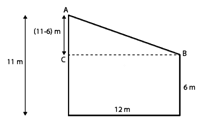The distance between points A and B is the distance between the tops of the poles.

The points A, B, and C form a right triangle, with AB as the hypotenuse, as seen in the diagram.

By using the Pythagoras Theorem in △ABC,

(11−6)+ 12= AB2

AB2 = 25 + 144

AB2 = 169

AB = 13

So, the distance between the tops of the poles is 13 m.

Question 8. A man goes 15 m due west and then 8 m due north. How far is he from the starting point?

Solution 8:If a man travels 15 meters west and then 8 meters north,

Let O represent the starting point and P represent the ending point.

The hypotenuse of the triangle is then OP.

We can find the distance OP using Pythagoras' theorem.

OP2 = 15+ 82

OP2 = 225 + 64

OP2 = 289

OP = 17

Hence, the required distance is 17 m.

Question 9. The foot of a ladder is 6 m away from a wall and its top reaches a window 8 m above the ground. If the ladder is shifted in such a way that its foot is 8 m away from the wall, to what height does its top reach?

Solution 9: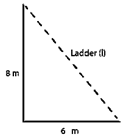By using the Pythagoras theorem, the length of the ladder is = L2

6+ 8= L2

L2 = 36 + 64 =100

L = 10

Thus, the length of the ladder is 10 m.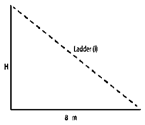Let the height of the ladder after it is shifted be H m.

By using the Pythagoras theorem, we can find the height of the ladder after it is shifted.

8+ H= 102

H= 100 – 64 = 36

H = 6

Thus, the height of the ladder is 6 m.

Question 10. A ladder 50 dm long when set against the wall of a house just reaches a window at a height of 48 dm. How far is the lower end of the ladder from the base of the wall?

Solution 10: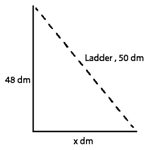In the question given that length of a ladder is 50dm

Let the distance of the lower end of the ladder from the wall be x dm.

By using the Pythagoras theorem,

x2 + 48= 502

x2 = 50− 48

= 2500 – 2304

= 196

H = 14 dm

Hence, the distance of the lower end of the ladder from the wall is 14 dm.

Question 11. The two legs of a right triangle are equal and the square of the hypotenuse is 50. Find the length of each leg.

Solution 11:

According to the Pythagoras theorem,

(Hypotenuse)2 = (Base)2 + (Height)2

Given that the square of the hypotenuse is 50 and the two legs of a right triangle are equal,

Each leg of the given triangle should be x units long.

Using Pythagoras' theorem

x+ x= (Hypotenuse)2

x+ x= 50

2x= 50

x2 = 25

x = 5

Hence, the length of each leg is 5 units.

Question 12. Verity that the following numbers represent Pythagorean triplet:

(i) 12, 35, 37

(ii) 7, 24, 25

(iii) 27, 36, 45

(iv) 15, 36, 39

Solution 12:

(i) The square of the largest side must equal the number of the squares of the other two sides in a Pythagorean triplet.

372 =1369

122 + 352 = 144 + 1225 = 1369

122 + 352 = 372

Yes, they represent a Pythagorean triplet.

(ii) The square of the largest side must equal the number of the squares of the other two sides in a Pythagorean triplet.

25= 625

72 + 24= 49 + 576 = 625

72 + 242 = 252

Yes, they represent a Pythagorean triplet.

(iii) The square of the largest side must equal the number of the squares of the other two sides in a Pythagorean triplet.

45= 2025

272 + 362 = 729 + 1296 = 2025

272 + 362 = 452

Yes, they represent a Pythagorean triplet.

(iv) The square of the largest side must equal the number of the squares of the other two sides in a Pythagorean triplet.

392 = 1521

15+ 362 = 225 + 1296 = 1521

152 + 362 = 392

Yes, they represent a Pythagorean triplet.

Question 13. In △ABC, ∠ABC = 100°, ∠BAC = 35° and BD ⊥ AC meets side AC in D. If BD = 2 cm, find ∠C, and length DC.

Solution 13: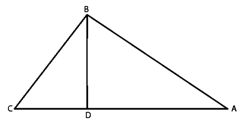As we know the sum of all angles of a triangle is 180o

Thus, for the given △ABC,

∠ABC + ∠BAC + ∠ACB = 180o

100o + 35o + ∠ACB = 180o

∠ACB = 180o –135o

∠ACB = 45o

∠C = 45o

By applying same way for the △BCD,

∠BCD + ∠BDC + ∠CBD = 180o

45o + 90o + ∠CBD = 180o (∠ACB = ∠BCD and BD is perpendicular to AC)

∠CBD = 180– 135o

∠CBD = 45o

The sides opposite to equal angles have equal length.

Thus, BD = DC

DC = 2 cm

Question 14. In a △ABC, AD is the altitude from A such that AD = 12 cm. BD = 9 cm and DC = 16 cm. Examine if △ABC is right angled at A.

Solution 14: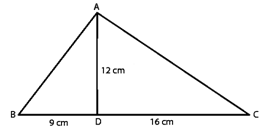Using the Pythagoras theorem,

12+ 16= AC2

AC2 = 144 + 256

= 400

AC = 20 cm

Using the Pythagoras theorem,

12+ 9= AB2

AB= 144 + 81 = 225

AB = 15 cm

For △ABC,

BC2 = 252 = 625

AB2 + AC2 = 152 + 20= 625

AB2 + AC2 = BC2

Because it satisfies the Pythagoras theorem, therefore △ABC is right angled at A.

Question 15. Draw a triangle ABC, with AC = 4 cm, BC = 3 cm and ∠C = 105o. Measure AB. Is (AB)= (AC) + (BC)2? If not which one of the following is true:

(AB)2 > (AC)2 + (BC)2 or (AB)< (AC)+ (BC)2?

Solution 15: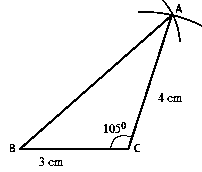Draw △ABC as shown in the figure according to following steps.

Draw a line BC = 3 cm.

At point C, draw a line at 105o angle with BC.

Take an arc of 4 cm from point C, which will cut the line at point A.

Now, join AB, which will be 5.5 cm.

AC+ BC2 = 42 + 3

= 9 + 16

= 25

AB2 = 5.52 = 30.25

AB2 is not equal to AC2+ BC2

Thus,  AB2 > AC+ BC2

Question 16. Draw a triangle ABC, with AC = 4 cm, BC = 3 cm and ∠C = 80°. Measure AB. Is (AB)= (AC) + (BC)2? If not which one of the following is true:

(AB)> (AC)2 + (BC)2 or (AB)< (AC)+ (BC)2?

Solution 16:Draw △ABC as shown in the figure according to following steps.

Draw a line BC = 3 cm.

At point C, draw a line at 80° angle with BC.

Take an arc of 4 cm from point C, which will cut the line at point A.

Now, join AB, it will be approximately 4.5 cm.

AC2 + BC2 = 42 + 32

= 9 +16

= 25

AB2 = (4.5)2

= 20.25

AB2 not equal to AC+ BC2

Thus, AB< AC+ BC2